# Gravity

## Gravity

The following text is used only for teaching, research, scholarship, educational use and informative purpose following the fair use principles.

We thank the authors of the texts and the source web site that give us the opportunity to share their knowledge

# Gravity

Newton’s Law of Gravitation*

states that any two point masses in the universe attract each other with a force that is directly proportional to the product of their masses, and inversely proportional to the square of the distance between them.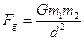G (known as ‘big G’) is a constant: its value is 6.7 x 10-11 N m2 kg-2 (you don’t need to know this).

Look over Worked Problems 10 and 11, page 114 and then try Exercise 10.5, page 115, Questions 1 – 6.

Gravity and Weight

We know that weight and mass are related by the following formula:

W = mg

Weight (as we have seen from the chapter on Force) is merely a shorthand way of saying force due to gravity.

Therefore we could re-write W = mg, as        Fg = mg            {where Fg represents force due to gravity}

But we have just seen above thatWe can equate both equations to get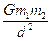=  mg
Now on the left hand side one of these masses (m1) represents the mass of the Earth (or any other planet).
m2 represents the mass of a second object, while on the right hand side m also represents the mass of this object.
So cancelling this m on both sides (corresponding to the mass of the object) we get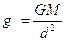This lets us calculate acceleration due to gravity (g) at any distance from a given planet.

Leaving Cert Physics Syllabus

 Content Depth of Treatment Activities STS Gravity Newton’s law of universal gravitation.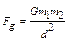Compare gravitational forces between Earth and Sun and between Earth and Moon. Solar System. Weight = mg Variation of g, and hence W, with distance from centre of Earth Appropriate calculations. “Weightlessness” and artificial gravity. Value of acceleration due to gravity on other bodies in space, e.g. Moon. Calculation of weight on different planets. Presence of atmosphere.

## *Newton’s Law of Gravitation

States that any two point masses in the universe attract each other with a force that is directly proportional to the product of their masses, and inversely proportional to the square of the distance between them.
Mathematically:                      FG  µ  (M1 M2),

And                                          F µ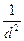Putting this together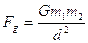Where G is a constant, as mentioned above.

This means that each of us is ‘attracted’ to every one of our colleagues, and the bigger they are, the greater is our attraction!
Contrary to what you may have thought, it also means that you are more likely to be attracted to your average American than to a person of any other nationality on this planet (Samoans excepted).
So there.

Physics and Astrology
We can use this law to test one of the fundamental concepts in astrology; how strong is the (gravitational) influence of a planet (or the moon) on a new-born baby compared to the influence of a near-by building?
Stick the numbers into the formula above and see for yourself!

Physics and Chaos
So we can use this to predict the motion of any planet due to the existence of another neighbouring planet. The next challenge was to see if it was possible to come up with an equation which would allow for the motion of a planet due to existence of two other planets.
Scientists quickly realised that this was an incredibly complex problem and was impossible to solve with the maths tools available to them at that time.
It was one of the first examples of what we now call Chaos Theory. The underlying rules were simple but the process rapidly becomes very complex. Scientists have made a lot of progress in this area since the advent of the computer. There is a wonderful documentary (available on YouTube) called ‘The Colours of Infinity’ which explains Chaos Theory much better than I ever could. By the way, Chaos Theory explains why the weather can never be predicted more than 13 days in advance, regardless of what local experts will tell you.

## Hooke and Newton

Robert Hooke (remember Hooke’s Law?) was probably Britain’s foremost scientist (or ‘natural philosopher’ as they were then known) when Newton first came to prominence. Over the following years the men became bitter enemies, to the extent that when Newton replaced Hooke as president of the Royal Society in England, the painting of Hooke which had pride of place in the building mysteriously went ‘missing’ shortly after Newton replaced him.

One reason they fell out was because Hooke was a mechanist. He believed that it was the scientist’s job to engage with nature’s causes – the cause of light, of gravity and so on – and how these causes produce effects that we can detect with instruments. Newton, on the other hand, saw physics as concerned with the mathematical expression of effects. For Newton the true causes of nature, being divine, were beyond the business of science.
The truth is, Newton could describe gravity, but he didn’t know how it worked. “Gravity must be caused by an agent acting constantly according to certain laws,” he admitted. “But whether this agent be material or immaterial, I have left to the consideration of my readers.”

After Newton died scientists went to a lot of trouble to promote him as the person to whom all other scientists should aspire. So they downplayed his interest in both astrology and alchemy. They would also have been uncomfortable with the role which he believed God played in maintaining the motion of the heavens. Sometimes they went a little overboard. One Victorian biographer noted that in addition to his intellectual contribution, Newton’s life was ‘one continual source of labour, patience, charity, generosity, piety, goodness and all other virtues, without a mixture of any vice whatsoever’.
Hmmm. . .
Nevertheless, as I go on to explain in another chapter (Circular Motion) Newton’s big achievement was to show that the motion of the heavens did actually follow rules of Physics and so could be predicted. It was this which later led Einstein to comment that “the most incomprehensible fact about the world is that it is comprehensible”.

Exam Questions
Radius of the earth = 6.36 × 106 m

Acceleration due to gravity at the earth’s surface = 9.81 m s−2
Distance from the centre of the earth to the centre of the moon = 3.84 × 108 m

• [2008 OL]

State Newton’s law of universal gravitation.

• [2006 OL]

Give two factors which affect the size of the gravitational force between two bodies.

• 

What is the relationship between the acceleration due to gravity g and the distance from the centre of the earth?

• 
• The international space station (ISS) moves in a circular orbit around the equator at a height of 400 km. What type of force is required to keep the ISS in orbit?
• What is the direction of this force?

• [2008 OL]

Calculate the acceleration due to gravity on the moon.
The radius of the moon is 1.7 × 106 m and the mass of the moon is 7 × 1022 kg.

• 

Calculate the acceleration due to gravity at a point 400 km above the surface of the earth.
The radius of the earth is 6.4 × 106 m and the mass of the earth is 6.0 × 1024 kg.

• 

Calculate the acceleration due to gravity at a height above the surface of the earth, which is twice the radius of the earth.

• An astronaut in the ISS appears weightless.

Explain why.

• 

Describe the variation in the weight of the astronauts as they travel to the moon.

• [2003 OL]

Why is the acceleration due to gravity on the moon less than the acceleration due to gravity on the earth?

• [2005 OL]

The earth is covered with a layer of air called the atmosphere.
What holds this layer of air close to the earth?

• [2006 OL]

The earth is surrounded by a layer of air, called its atmosphere.
Explain why the moon does not have an atmosphere.

• At what height above the earth’s surface will the astronauts experience weightlessness?

Assume the mass of the earth is 81 times the mass of the moon.

• The moon orbits the earth every 27.3 days. What is its velocity, expressed in metres per second?

Exam Solutions

• Newton’s Law of Gravitation states that any two point masses in the universe attract each other with a force that is directly proportional to the product of their masses, and inversely proportional to the square of the distance between them.
• The mass of the objects and the distance between them.
• g is proportional to 1/d2
•
• Gravity
• Towards the centre of the orbit / inwards / towards the earth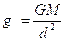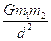•

=  mg         Þ

Þ g = (6.7 × 10–11)( 7 × 1022) / (1.7 × 106)2
Þ g = 1.6 m s-2

•= mg         Þ

note that d represents the distance from the point to the centre of the earth.
Þ g = (6.67 × 10–11)( 6.0 × 1024) / (400 000 + 6.4 × 106)2          Þ g = 8.6 m s-2

• Note that 2d above surface is 3d from earth’s centre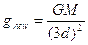where d = 6.36 × 106 m

gnew = 1.09 m s-2

• He is in a state of free-fall (the force of gravity cannot be felt).
• Weight decreases as the astronaut moves away from the earth and gains (a lesser than normal) weight as she/he approaches the moon
• The earth has a greater mass than the moon.
• Gravity.
• The gravitational force is too weak to sustain an atmosphere.
• Gravitational pull of earth = gravitational pull of moon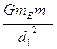=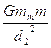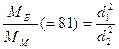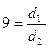dE = 9 dm and dE + dm = 3.84 × 108 m

10 dm = 3.84 × 108
dm = 3.84 × 107
dE = 3.356 × 108
Height above the earth = (3.356 × 108) – (6.36 × 106) = 3.39 × 108 m

•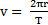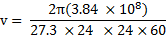v = 1022.9 m s-1

Source : http://www.thephysicsteacher.ie/LC%20Physics/Student%20Notes/10.2%20Gravity.doc

Author : not indicated on the source document of the above text

### Gravity

#### Gravity

Main page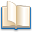Alimentation - nutritionAnimalsArchitectureArtAstrology, esoteric, parapsychology and fantasyAstronomyBiology and geneticsBotany and agricultureChemistryCourses, training, guides, handbooks and tipsCulture General and actualityDermatology, health and wellnessDictionaries and glossariesEconomics and financeEducation and pediatricsEngineering and technologyEnglish grammar and anthologyFashion and show businessFitnessFood, recipes and drinkGames, play spaceGeography, geology and environmentHistoryInformatics and computer worldJobs worldLanguagesLeisure and DIY do it yourselfLessons for studentsLiterature, biographies and journalismMathematics, geometry and statisticalMedicineMusicNotes and concept mapsPhilosophyPhotography and images - picturesPhysicsPhysiologyPsychology and psychoanalysisQuiz questions and answersReligionsRights law and political scienceScienceSociology and cultural anthropologySportSummaries and reviewsTelevision, film - movies and comicsTheater and communicationsTheses, themes and dissertationsTravel and tourist guidesUseful documents and tablesUtility, calculators and convertersWeather and meteorology

#### Gravity

This is the right place where find the answers to your questions like :

Who ? What ? When ? Where ? Why ? Which ? How ? What does Gravity mean ? Which is the meaning of Gravity?

##### Gravity physics notes

Alanpedia.com from 1998 year by year new sites and innovations